SSC BOARD PAPERS IMPORTANT TOPICS COVERED FOR BOARD EXAM 2024

### Solve the following problems: Equal heat is given to two objects A and B of mass 1 g. Temperature of A increases by 3oC and B by 5oC. Which object has more specific heat? And by what factor?

Question 14.

Solve the following problems:

Equal heat is given to two objects A and B of mass 1 g. Temperature of A increases by 3oC and B by 5oC. Which object has more specific heat? And by what factor?

Specific heat is the amount of heat per unit mass required to raise the temperature by one degree Celsius.

It is given by the formula:

Q = mcΔT

Or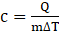Where, Q is the amount of heat added.

T is the temperature.

“c” is the specific heat

“m” is the mass

Now, let say, two objects A and B.

Then, the ratio of the specific heats of two objects is given as: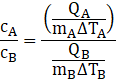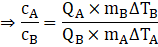As per the question, equal heat is given to two objects A and B of mass 1 g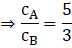Or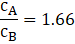Clearly, it shows that the specific heat of A is greater than B with a factor 1.66.